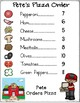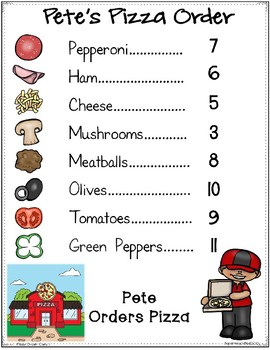# Real-Life Word Problems Pete Adds Pizza Orders 11 - 20Subject
Resource Type
File Type
PDF (9 MB|30 pages)
Standards
\$6.50
Also included in:
1. Addition Real-Life Word Problems BUNDLE 1-20 This product is a bundled product of Annie Adds Lunch Count and Pete Adds Pizza: Real Life Word Problems. Purchase these together here you save money when compared to purchasing these products separately. This resource is a flexible small group or inde
\$13.00
\$9.10
Save \$3.90
• Product Description
• Standards
This product is part of bundle which you will save you money when purchased together with Annie Adds Lunch Count

This resource is a flexible small group or independent work station/center
activity to practice interpreting information from a pizza topping order chart,
using that information to construct addition problems for numbers 11 – 20.
Students add the pizza topping items his friends want listed on the chart.
Using task cards asking “how many” and addends and sums from 11 – 20, students work out the simple addition problems using the pizza topping tokens and/or number cards
and record them on the task cards.

The set of 20 task cards provides the task cards in 3 differentiated levelsstyle='mso-spacerun:yes'> (text, text + picture, and picture) to make it easy to use in your classroom. Each set is designated by different symbol in the bottom left corner of each card to facilitate sorting. Use all the cards or
just some of the set to suit the needs of your students.

You also have the option of using the vertical and horizontal equation charts for
students to lay out their problems for better visualization.

WHAT YOU GET
⭐5 different Pete’s Pizza Order cards
⭐3 leveled sets each with 20 task cards
⭐Number cards 0 – 20
⭐10 picture pizza cards of each item (pepperoni, ham, cheese, mushrooms, meatballs, olives, tomatoes and green peppers).

STANDARDS COVERED
1. Given a number from 11-20,
as 10 things arranged in an array,
2. Given a number from 11-20, count out that many objects and add them to find the
sum.
4. Represent and solve problems involving addition.
5. Use addition 10 to solve word problems involving situations of adding to, putting
together, and comparing, by using objects, drawings, and equations with a
symbol for the unknown number to represent the problem.
PREP FOR USE
1.Following printing, cut task cards sheets in half.
2. Cut out number cards and pizza topping cards.
3. You can laminate if you choose and add Velcro to task cards and number
cars if desired.

Related Products
Number 1-9 Numeral Cards with Touch Points
Nickel Coin Cup Matching Task Cards 5-50 Cents FREEBIE
More or Less Than Number Game {Common Core}

If at any time you have questions or concerns, please don’t hesitate to contact
me at
You can also find me at SpecialEdSpot.com
and
Fluently add and subtract within 20 using mental strategies. By end of Grade 2, know from memory all sums of two one-digit numbers.
Use addition and subtraction within 100 to solve one- and two-step word problems involving situations of adding to, taking from, putting together, taking apart, and comparing, with unknowns in all positions, e.g., by using drawings and equations with a symbol for the unknown number to represent the problem.
Determine the unknown whole number in an addition or subtraction equation relating three whole numbers. For example, determine the unknown number that makes the equation true in each of the equations 8 + ? = 11, 5 = ▯ - 3, 6 + 6 = ▯.
Understand the meaning of the equal sign, and determine if equations involving addition and subtraction are true or false. For example, which of the following equations are true and which are false? 6 = 6, 7 = 8 - 1, 5 + 2 = 2 + 5, 4 + 1 = 5 + 2.
Add and subtract within 20, demonstrating fluency for addition and subtraction within 10. Use strategies such as counting on; making ten (e.g., 8 + 6 = 8 + 2 + 4 = 10 + 4 = 14); decomposing a number leading to a ten (e.g., 13 - 4 = 13 - 3 - 1 = 10 - 1 = 9); using the relationship between addition and subtraction (e.g., knowing that 8 + 4 = 12, one knows 12 - 8 = 4); and creating equivalent but easier or known sums (e.g., adding 6 + 7 by creating the known equivalent 6 + 6 + 1 = 12 + 1 = 13).
Total Pages
30 pages